# The Square Antiprism

The square antiprism is a 3D uniform polyhedron bounded by 10 polygons (2 squares and 8 triangles), 16 edges, and 8 vertices.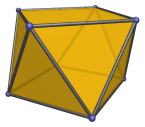The height of a square antiprism with an edge length of 2 is 23/4, or approximately 1.68179.

A square antiprism can be capped with a square pyramid to form the gyroelongated square pyramid (J10), a Johnson solid, or with two square pyramids to form the gyroelongated square bipyramid (J17), another Johnson solid.

The dual of the square antiprism is the square trapezohedron.

## Projections

In order to be able to identify the square antiprism in various projections of 4D objects, it is useful to know how it appears from various viewpoints. The following are some of the commonly-encountered views:

Projection Envelope Description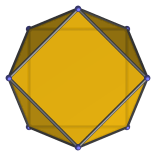Regular octagon

Parallel projection centered on square face.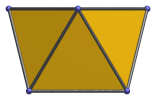Trapezium

Parallel projection parallel to square faces and a pair of triangles.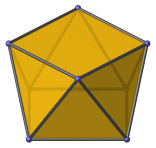Pentagon

Vertex-centered parallel projection.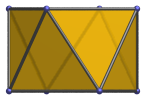Rectangle

Parallel projection centered on an edge between two triangles.

## Coordinates

The Cartesian coordinates of the square antiprism, centered on the origin and having edge length 2, are:

• (0, ±√2, 1/∜2)
• (±√2, 0, 1/∜2)
• (±1, ±1, −1/∜2)

## Occurrences

The square antiprism occurs in the following CRF polytopes:

Last updated 10 Jan 2023.